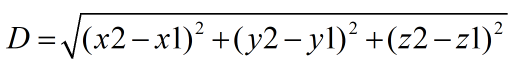Request a Tool

Distance Between 2 Points Calculator

Distance Between 2 Points Calculator is use to calculate distance between two points.

Distance
0

Formula• D = Distance
• x1 = Value of x1
• x2 = Value of x2
• y1 = Value of y1
• y2 = Value of y2
• z1 = Value of z1
• z2 = Value of z2

Defination / Uses

The length of the line segment connecting two places is called the distance between them. The length of the line segment connecting the specified coordinates can be used to compute the distance between two points in coordinate geometry.

There is exactly one line segment connecting any two points. The length of the line segment connecting two points is the distance between them. It's important to keep in mind that the distance between two points is always positive. Congruent segments are segments that are the same length. The endpoints are usually used without an overline to indicate the length of a segment. The length of AB, for example, is denoted by A B or sometimes A B. When determining the distance between two points, a ruler is commonly used. The distance between two points is calculated by placing the 0 mark at the left endpoint and the mark on which the other endpoint falls.